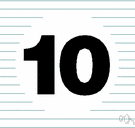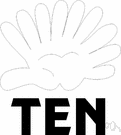ten

(redirected from Ten (disambiguation))
Also found in: Thesaurus, Medical, Encyclopedia, Wikipedia.

ten

(tĕn)
n.
1. The cardinal number equal to 9 + 1.
2. The tenth in a set or sequence.
3. Something having ten parts, units, or members.
4. Games A playing card marked with ten spots.
5. A ten-dollar bill.

[Middle English, from Old English tīen; see dekm̥ in Indo-European roots.]

ten

(tɛn)
n
1. (Mathematics) the cardinal number that is the sum of nine and one. It is the base of the decimal number system and the base of the common logarithm. See also number1
2. (Mathematics) a numeral, 10, X, etc, representing this number
3. something representing, represented by, or consisting of ten units, such as a playing card with ten symbols on it
4. Also called: ten o'clock ten hours after noon or midnight
determiner
a. amounting to ten: ten tigers.
b. (as pronoun): to sell only ten.
[Old English tēn; related to Old Saxon tehan, Old High German zehan, Gothic taihun, Latin decem, Greek deka, Sanskrit dasa]

ten

(tɛn)

n.
1. a cardinal number, nine plus one.
2. a symbol for this number, as 10 or X.
3. a set of this many persons or things.
4. a ten-dollar bill.
5. Also called ten's place.
a. (in a mixed number) the position of the second digit to the left of the decimal point.
b. (in a whole number) the position of the second digit from the right.
6. amounting to ten in number.
[before 900; Middle English ten(e), tenn(e), Old English tēn(e), tīen(e), c. Old Frisian tian, Old Saxon tehan, Old High German zehan, Old Norse tīu, Gothic taihun, Latin decem, Greek déka, Skt daśa]

ten.

1. tenor.
2. Music. tenuto.
ThesaurusAntonymsRelated WordsSynonymsLegend:
 Noun 1ten - the cardinal number that is the sum of nine and one; the base of the decimal systemlarge integer - an integer equal to or greater than ten 2 ten - one of four playing cards in a deck with ten pips on the faceten-spotspot - a playing card with a specified number of pips on it to indicate its value; "an eight-spot" Adj. 1ten - being one more than nine    10, xcardinal - being or denoting a numerical quantity but not order; "cardinal numbers"

ten

determiner
Related words
prefix deca-
Translations
tien
الرَّقْم 10العاشِرعشرةعَشَرَةعُمْر العاشِرَه
deu
desetdeset letdesetiletý
ti
dek
kümme
kymmenen
deset
tíztizes
sepuluh
tíutíu ára
10
decem
dešimtdešimtasdešimtasisdešimtiesdešimties metų
zece
desaťdesať rokov
desetdesetica
tio
kumi
สิบ
mười

ten

[ten]
B. Ndiez m
tens of thousandsdecenas de miles
ten to one he'll be late
they're ten a penny
see five for usage

ten

[ˈtɛn] numdix
She's ten → Elle a dix ans.
tens of thousands → des dizaines fpl de milliers

ten

adjzehn; the Ten Commandmentsdie Zehn Gebote; ten to one he won’t come(ich wette) zehn gegen or zu eins, dass er nicht kommt; a packet of ten (cigarettes)eine Zehnerpackung (Zigaretten)
nZehn f; tens (Math) → Zehner pl; to count in tens; you can only buy them in tensman kann sie nur in Zehnerpackungen kaufen; Lynn’s a ten (inf: = very nice) → Lynn ist ’ne Nummer 10 (inf), → Lynn ist perfekt ? also six

ten

:
tenpence
nzehn Pence; (also tenpenny piece)Zehnpencestück nt
tenpin bowling, (US) tenpins
nBowling nt

ten

[tɛn]
2. ndieci m inv
tens of thousands →
ten to one he'll be late (fam) → dieci a uno che arriva tardi
they're ten a penny (fam) → ce ne sono a bizzeffe
for usage see five

ten

(ten) noun
1. the number or figure 10.
2. the age of 10.
1. 10 in number.
2. aged 10.
ten-
having ten (of something). a ten-pound fine.
tenth noun
1. one of ten equal parts.
2. (also adjective) the last of ten (people, things etc); the next after the ninth.
ten-pin bowling noun
a game in which a ball is rolled at ten skittles in order to knock down as many as possible.
ˈten-year-old noun
a person or animal that is ten years old.